﻿ Python与Echarts相结合的可视化工具：pyecharts – 程序圆教程网# Python与Echarts相结合的可视化工具：pyecharts

pyecharts是一款将python与echarts结合的强大的数据可视化工具，本文将为你阐述pyecharts的使用细则。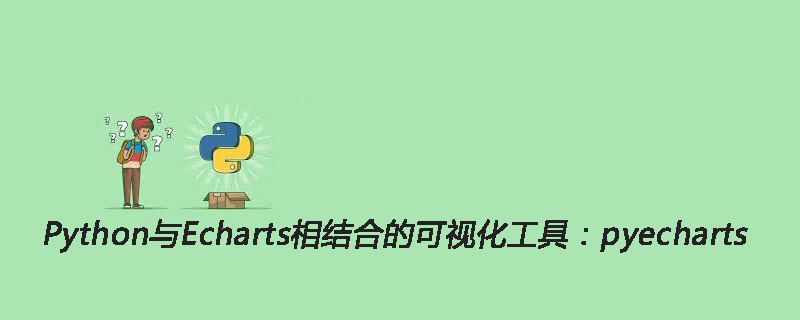`pip install pyecharts`

pip install -i https://pypi.tuna.tsinghua.edu.cn/simple pyecharts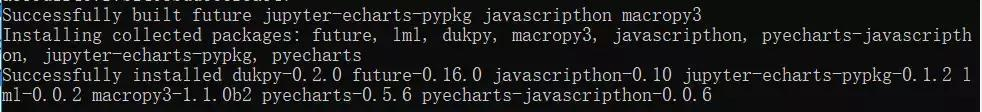`from __future__ import unicode_literals`

```//导入柱状图-Bar
from pyecharts import Bar
//设置行名
columns = ["Jan", "Feb", "Mar", "Apr", "May", "Jun", "Jul", "Aug", "Sep", "Oct", "Nov", "Dec"]
//设置数据
data1 = [2.0, 4.9, 7.0, 23.2, 25.6, 76.7, 135.6, 162.2, 32.6, 20.0, 6.4, 3.3]
data2 = [2.6, 5.9, 9.0, 26.4, 28.7, 70.7, 175.6, 182.2, 48.7, 18.8, 6.0, 2.3]
//设置柱状图的主标题与副标题
bar = Bar("柱状图", "一年的降水量与蒸发量")
//添加柱状图的数据及配置项
bar.add("降水量", columns, data1, mark_line=["average"], mark_point=["max", "min"])
bar.add("蒸发量", columns, data2, mark_line=["average"], mark_point=["max", "min"])
//生成本地文件（默认为.html文件）
bar.render()```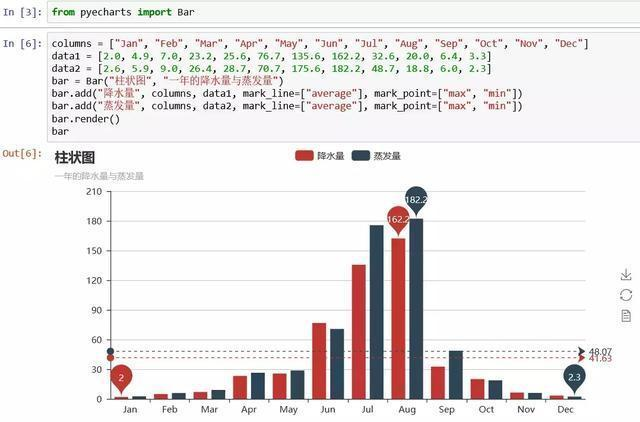```//导入饼图Pie
from pyecharts import Pie
//设置主标题与副标题，标题设置居中，设置宽度为900
pie = Pie("饼状图", "一年的降水量与蒸发量",title_pos='center',width=900)
//加入数据，设置坐标位置为【25，50】，上方的colums选项取消显示
//加入数据，设置坐标位置为【75，50】，上方的colums选项取消显示，显示label标签
//保存图表
pie.render()```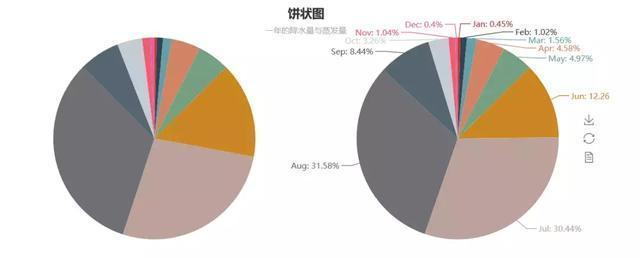```//导入箱型图Boxplot
from pyecharts import Boxplot
boxplot = Boxplot("箱形图", "一年的降水量与蒸发量")
x_axis = ['降水量','蒸发量']
y_axis = [data1,data2]
//prepare_data方法可以将数据转为嵌套的 [min, Q1, median (or Q2), Q3, max]
yaxis = boxplot.prepare_data(y_axis)
boxplot.render()```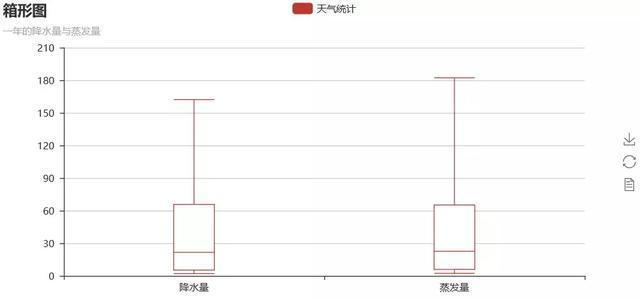```from pyecharts import Line
line = Line("折线图","一年的降水量与蒸发量")
//is_label_show是设置上方数据是否显示
line.render()```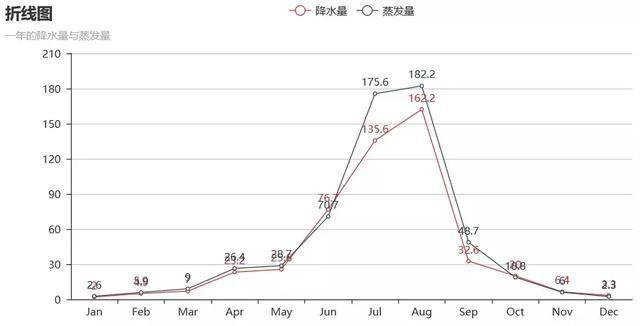```from pyecharts import Radar
//由于雷达图传入的数据得为多维数据，所以这里需要做一下处理
radar_data1 = [[2.0, 4.9, 7.0, 23.2, 25.6, 76.7, 135.6, 162.2, 32.6, 20.0, 6.4, 3.3]]
radar_data2 = [[2.6, 5.9, 9.0, 26.4, 28.7, 70.7, 175.6, 182.2, 48.7, 18.8, 6.0, 2.3]]
//设置column的最大值，为了雷达图更为直观，这里的月份最大值设置有所不同
schema = [
("Jan", 5), ("Feb",10), ("Mar", 10),
("Apr", 50), ("May", 50), ("Jun", 200),
("Jul", 200), ("Aug", 200), ("Sep", 50),
("Oct", 50), ("Nov", 10), ("Dec", 5)
]
//传入坐标
//一般默认为同一种颜色，这里为了便于区分，需要设置item的颜色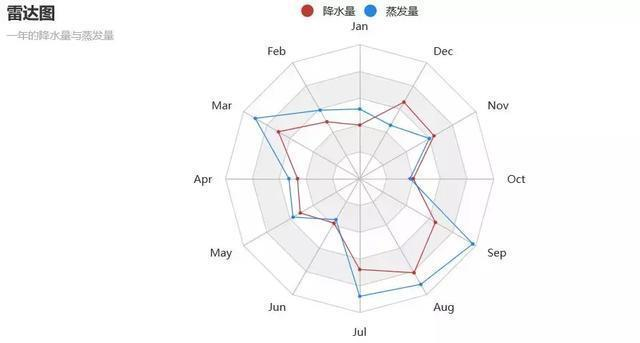```from pyecharts import Scatter
scatter = Scatter("散点图", "一年的降水量与蒸发量")
//xais_name是设置横坐标名称，这里由于显示问题，还需要将y轴名称与y轴的距离进行设置
yaxis_name_gap=40)
scatter.render()```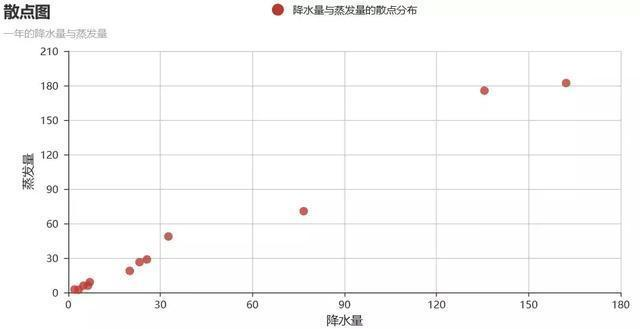```from pyecharts import Grid
//设置折线图标题位置
line = Line("折线图","一年的降水量与蒸发量",title_top="45%")
grid = Grid()
//设置两个图表的相对位置
grid.render()```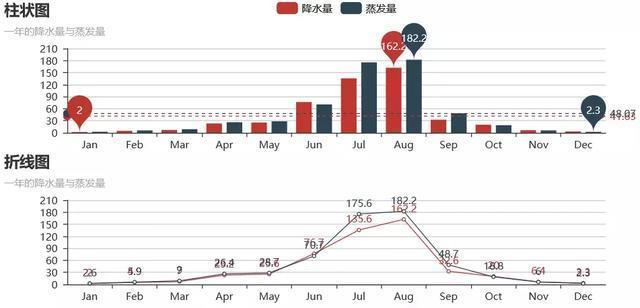```from pyecharts import Overlap
overlap = Overlap()
bar = Bar("柱状图-折线图合并", "一年的降水量与蒸发量")
overlap.render()```

（1）导入相关图表包

（2）进行图表的基础设置，创建图表对象

Python教程

## Python可视化神器：Plotly Express库

2021-4-27 8:10:46

Python教程

## Python如何运用turtle绘制阴阳太极图

2021-4-27 8:10:59

0 条回复 A文章作者 M管理员
暂无讨论，说说你的看法吧